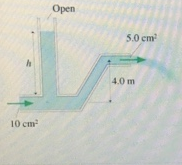# Problem: Water flows from the pipe shown in the figure with a speed of 8.0 m/s. (Figure 1)What is the water pressure as it exits into the air? Express your answer to two significant figures and include the appropriate units.

###### FREE Expert Solution

The pressure at the exit is equal to atmospheric pressure. Thus, water pressure at the exit is equal to atmospheric pressure.

84% (450 ratings)###### Problem Details

Water flows from the pipe shown in the figure with a speed of 8.0 m/s. (Figure 1)What is the water pressure as it exits into the air? Express your answer to two significant figures and include the appropriate units.